### IMO Shortlist 2015 problem G2

Kvaliteta:
Avg: 0,0
Težina:
Avg: 6,0

Triangle$ABC$ has circumcircle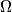$\Omega$ and circumcenter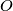$O$. A circle$\Gamma$ with center$A$ intersects the segment$BC$ at points$D$ and$E$, such that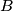$B$,$D$,$E$, and$C$ are all different and lie on line$BC$ in this order. Let$F$ and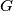$G$ be the points of intersection of$\Gamma$ and$\Omega$, such that$A$,$F$,$B$,$C$, and$G$ lie on$\Omega$ in this order. Let$K$ be the second point of intersection of the circumcircle of triangle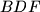$BDF$ and the segment$AB$. Let$L$ be the second point of intersection of the circumcircle of triangle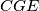$CGE$ and the segment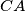$CA$.

Suppose that the lines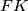$FK$ and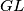$GL$ are different and intersect at the point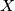$X$. Prove that$X$ lies on the line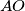$AO$.

(Greece)

Izvor: https://www.imo-official.org/problems/IMO2015SL.pdf